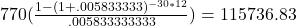## Rounding in the calculation of monthly interest rates is discouraged. Such rounding can lead to answers different from those presented here.

Question

Rounding in the calculation of monthly interest rates is discouraged. Such rounding can lead to answers different from those presented here. For long-term loans, the differences may be pronounced.

You find that the going rate for a home mortgage with a term of 30 years is 7% APR. The lending agency says that based on your income, your monthly payment can be at most \$770. How much can you borrow? (Round your answer to the nearest cent.)

in progress 0
2 weeks 2021-09-01T18:18:37+00:00 2 Answers 0 views 0

1. Step-by-step explanation:

115736.83

Step-by-step explanation:

Effective rate:

.07/12= .005833333333

770(\frac{1-(1+.005833333)^{-30*12}}{.005833333333})=115736.83770(

.005833333333

1−(1+.005833333)

−30∗12

)=115736.83